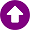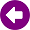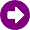﻿ Calculation method > ITU-R Rec. P.533

# ITU-R REC. P.533

Navegación:  Calculation method > ITU-R Rec. P.533

# ITU-R REC. P.533DESCRIPTION This model is based on the Recommendation 533 of the ITU-R and provides a method for the prediction of the performance of HF circuits which can be applied to links and coverage calculations. For a point to point link, it may exist various propagation modes, each one of them characterized by a determined number of hops in the ionosphere and by the layer (E or F2) in which the reflection occurs. For example, a determined path can be established by means of two different modes: one of them with three E layer hops and the other one with two F2 layer hops. The below shown calculations are done for every mode and then combined. The identification of the relevant modes depends on the link distance. DEVELOPMENT This prediction method consists of three parts. The first one evaluates the available frequencies for the link through estimating the basic MUF (Maximum Usable Frequency) of each propagation mode, the basic MUF of the path, which is the greater among all MUFs, and the probability that the operational MUF is lower than the MUF of the link, hence the circuit can be established. Given the ionosphere propagation characteristics, which depends on the different ionization grade, in this calculation method the Sun Spot Number or Wolf Number is required as data input, as well the date and time of the day of the prediction. In the second part of the process, the median field strength is calculated at reception points. For each possible mode, the basic transmission loss is computed combining the basic free space loss for the path, the reflection loss on the ground for modes with more than a single hop and the loss associated with different absorption phenomena in the ionosphere. From the field strength the received power is calculated for every propagation mode, and the total power is computed as the sum of all of them. At this stage of the calculation, the data of the equipment involved in the system are required, more precisely the transmission power and the antenna diagram and gain of the transmitter and receiver. In the third part, the link quality is evaluated computing the monthly median signal-to-noise ratio. When working in HF band, the dominant noise is captured with the external antenna, which is represented by an antenna noise factor Fa. This noise factor combines atmospheric noise and other sources as cosmic noise and thermal noise. The user should here indicate the type of environment for the man-made noise calculation. The options are: “city”, “residential”, ”rural”, “quiet-rural”, “quiet” and “noisy”. Then, a log-normal variation model is applied for the signal-to-noise ratio allowing to compute the probability to reach the specified threshold. The basic analogical circuit reliability is computed this way and it is compared with the reliability (%) objective that the user must supply as a reception parameter. In order to compute the digital circuit reliability, in addition to the signal-to-noise ratio, the next reception parameters are required: Time window, Tw (ms): The time interval within which signal modes will contribute to system performance and beyond which will reduce system performance. Frequency window, Fw (Hz): The frequency interval within which signal modes will contribute to system performance and beyond which will reduce system performance. Amplitude ratio, A (dB): For each propagating mode the hourly median value of the field strength will be predicted, taking account of transmitter power and of the antenna gain for that mode. The strongest mode at that hour will be determined and the amplitude ratio, A, is the ratio of the strength of the dominant mode to that of a sub-dominant mode, which will just affect the system performance if it arrives with a time delay beyond Tw or a frequency spread greater than Fw. REFERENCES  ITU-R Rec. P.533, "Method for the prediction of the performance of HF circuits"